# 3D Voronoi Diagrams and Medial Axis

6.838 May 11 Meeting 25

Marek Teichmann

 1. Definitions

### Voronoi Diagram of Point sites

• Set S of point sites
• Distance function: d(p,s) = Euclidean distance

Def: Voronoi Diagram

partition of space into regions VR(s) s.t.
For all p in VR(s),    d(p,s) < d(p,t) for all t not = s.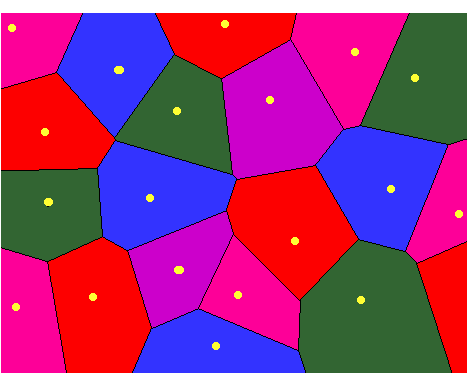## 2D:

• Voronoi polygons = Voronoi regions
• Voronoi edges (equidistant to 2 sites)
• Voronoi vertices (equidistant to 3 sites)

Alternative Def:

Given 2 sites s,t,
the bisector B(s,t) of s and t is the set of points p equidistant to s and t (generators)

Example: bisector of 2 points is a line.

Def: The dominance region of s over t is the set of points closer to s than t.Example: dominance region of a point in a 2 point set is a half-space.

Def: Voronoi region VR(s):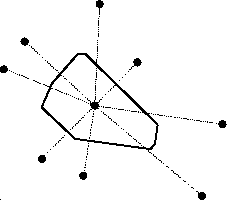Question: How to find a vertex of VR(s) ?

## In 3D:

• Voronoi regions = polytopes (convex polyhedron)
• Voronoi faces (bisectors, bound polytopes)
• Voronoi edges (intersections of faces)
• Voronoi vertices

Voronoi region of point in the center: (cross-eyed stereo pair)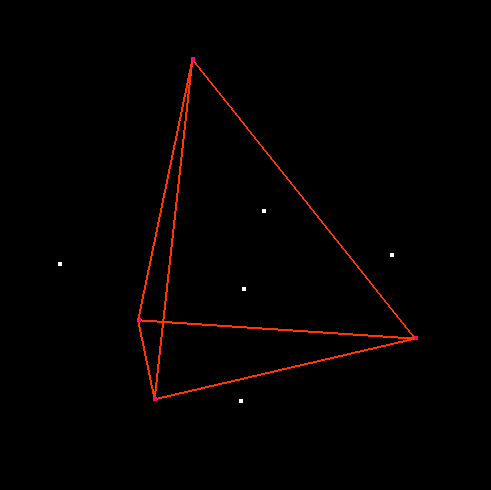## Construction:

DT  in 4D + duality (similar to 3D case)

• Map (x,y,z) --> (x,y,z, x2 + y2 + z2)
• Compute CONVEX - HULL  in R4.      Takes O(n2) time.
• Map to Delaunay triangulation in R3
• Tetrahedron circumsphere centers --> Voronoi vertices
• Tetrahedron faces --> Voronoi edges

## Alternate point of view (2D):

Front propagation / prairie fire analogy.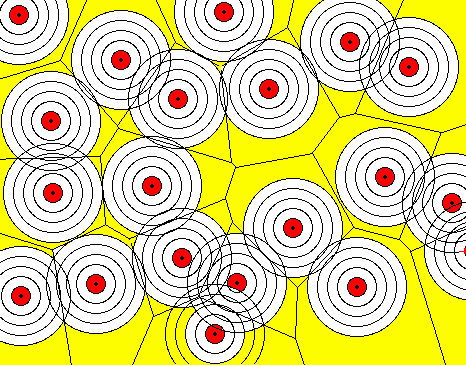(J. Smith, Oberlin).

Prairie fire analogy

At time t the front (fire) is a set of circles of radius t.
Circles intersect at Voronoi edges.

What is the relation to the distance function?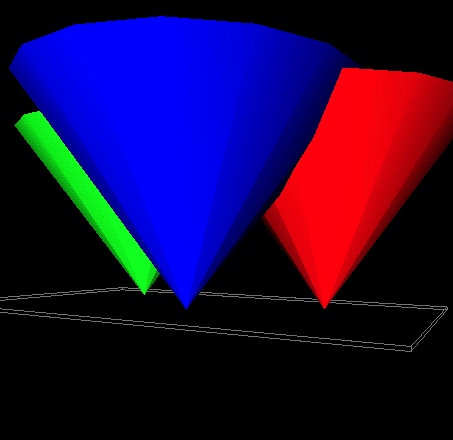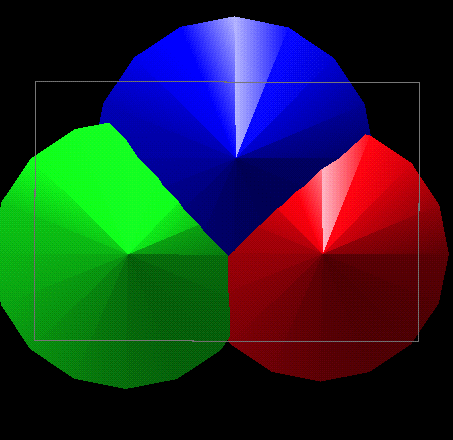Graph of distance function.

<demo: SceneViewer 3cones2.iv>

Voronoi edges = ridges of distance function.
Take min of all distance cones --> graph of distance function from all sites.

Generalizes to 3D.

 2. Voronoi diagram of polygons & Medial Axis

### General Definition: Medial Axis of a surface (curve)

Geometry in action page (Eppstein)

Set of points in space equidistant to 2 or more points on the surface (curve).

Examples:### Voronoi Diagram of polygon sites

• Set S of polygons
• Distance function: d(p,s) = Euclidean distance

## 2D:

Set of edges.

Bisectors: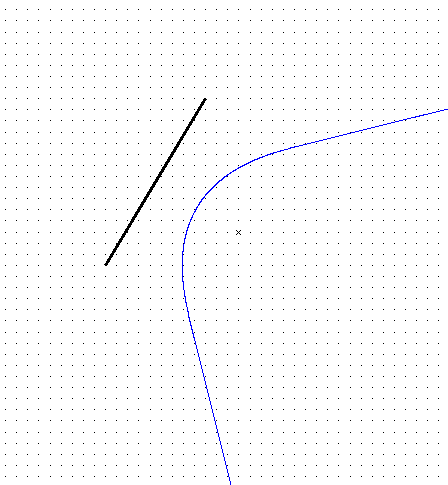Voronoi diagram: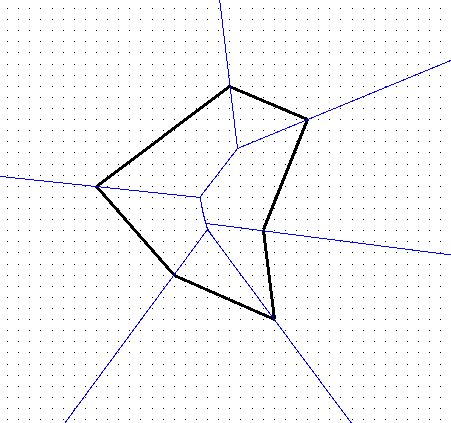Note: Voronoi diagram of convex polygon is linear.

Problem: Voronoi edges not well defined by distance functionSolution 1: Use bisector of edges as above.

Problem: How to do this in 3D?

Solution 2: Add vertices as sites: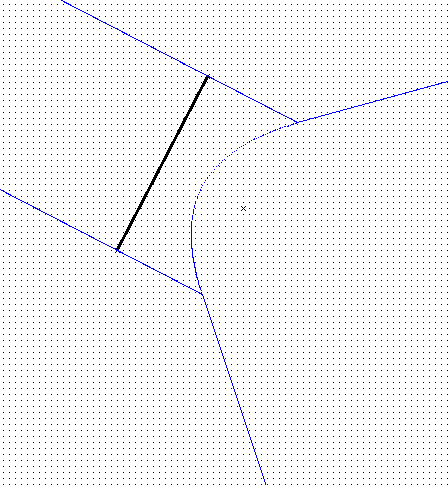Advantage: a bisector now has only ONE mathematical definition (formula).

Corresponding Voronoi diagram:Algorithm: incremental construction [Michael Seal, using LEDA]

HARD: why?Insertion of point b into Voronoi diagram of segments a and c

Intersection of quadratic curves,    exact arithmetic...

## Wavefront point of view• Wavefront meets at Voronoi edges
• Voronoi edges appear where wavefront changes from flat to circular (not in Medial Axis).

## Difference between Voronoi Diagram and Medial Axis

The medial axis is a subset of the Voronoi diagram of the edges and vertices of the polygon.
Voronoi edges that meet the reflex vertices are not part of the medial axis.

## 3D Voronoi Diagrams of polygons:

Bisectors:

 bisector type portion of point - point plane point - edge parabolic cylinder point - triangle paraboloid edge - edge hyperbolic paraboloid edge - triangle parabolic cylinder triangle - triangle plane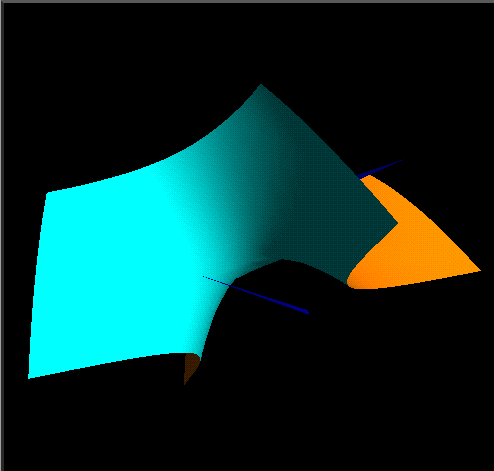Edges:

Curves of algebraic degree <= 4.Question: How to compute suface equidistant surface between 2 objects?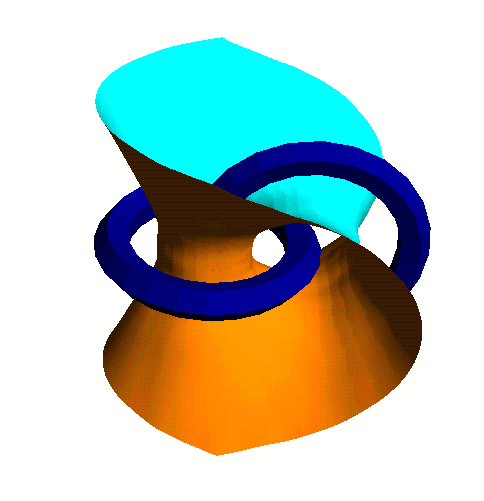<demo vor>

 Algorithms for VD/MA of polyhedral objects

No general exact algorithms for VD of triangles currently. Why?

--> use approximation algorithm!

## 0. Exact algorithm for convex case

Property: For convex polygonal objects, bisectors are linear.

Algorithm (based on wavefront idea):

• Input: set of planes: P1, ... Pn forming polyhedron
Equations: Pi:   ai x = bi   with ai unit.
• Build planes Qi at a distance t from Pi:    ai x + t = bi
• Consider Qi as planes in 4 dimensions.
• Take intersection of half-spaces  ai x + t <= bi
---> get graph of distance function (distance to all bounding planes Pi)
• Project back to R3 by eliminating t.

Running time O(n2).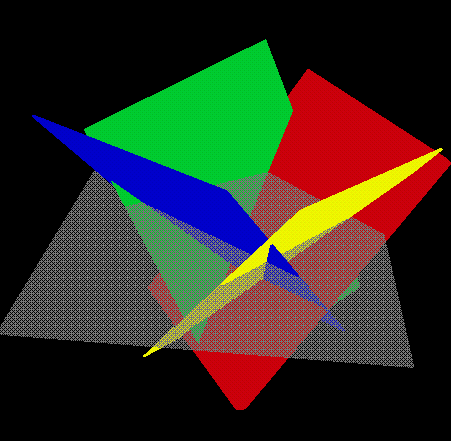## 1. Surface sampling + Voronoi diagram

• Sample surface of object uniformly
• Compute Voronoi diagram of samples
• Extract Medial Axis approximation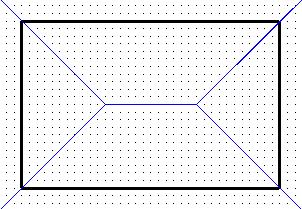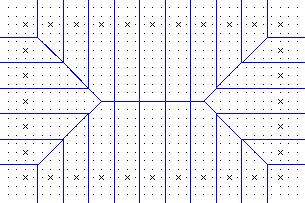<demo box>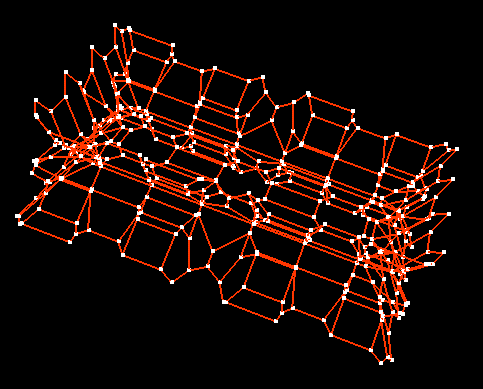## 2. Following ridges of distance function

Use numerical technique to follow curve where fronts meet.
Computes skeleton of medial axis (edges, vertices).

[E. Sherbrooke, N. Patrikalakis, Solid Modeling 1995]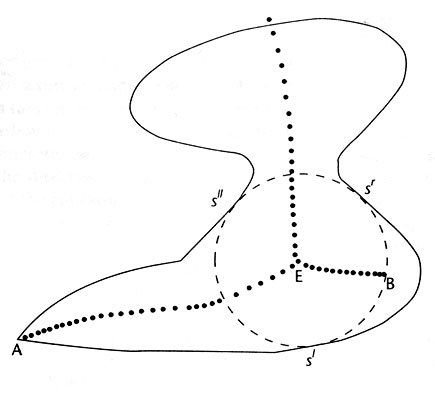Drawback?

## 3. Hierarchical voxel approximation

[Overmars et al.]

Idea:

• Divide space into cells (ex. voxels)
• Label cell corners by closest object (triangle)
• Identify cells containing Voronoi diagramProperty: Cell C intersects Voronoi diagram if number of labels L(C) > 1

Question: Is this an iff?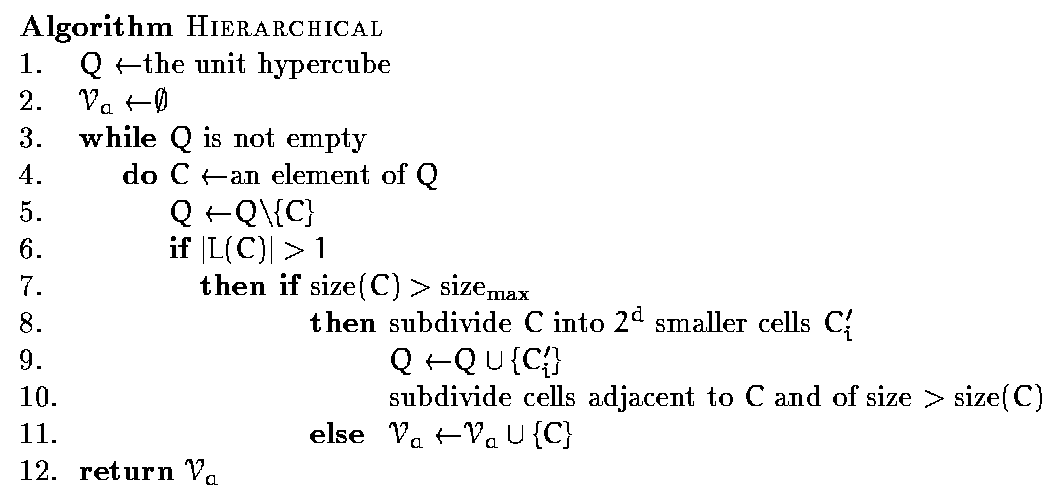Examples.

3d Voronoi diagram skeleton of a set of tetrahedra (stereo pair):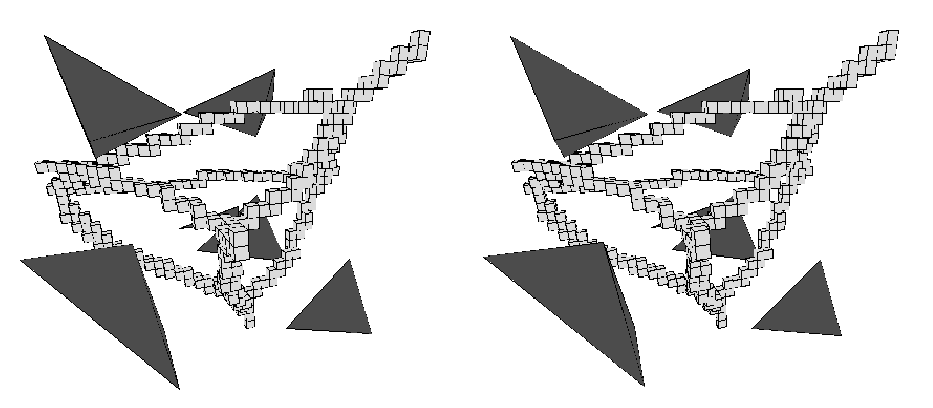2d example showing hierarchical subdivision: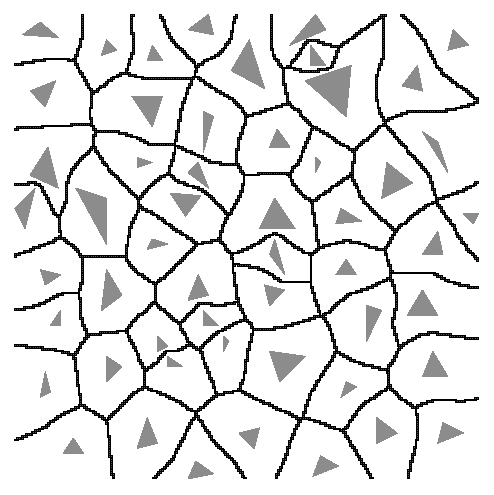## Cell Approximation with surface extraction

Use marching cubes-like algorithm for approximating Voronoi surfaces.

Use labels on corners:3D: Different cases for cell labels: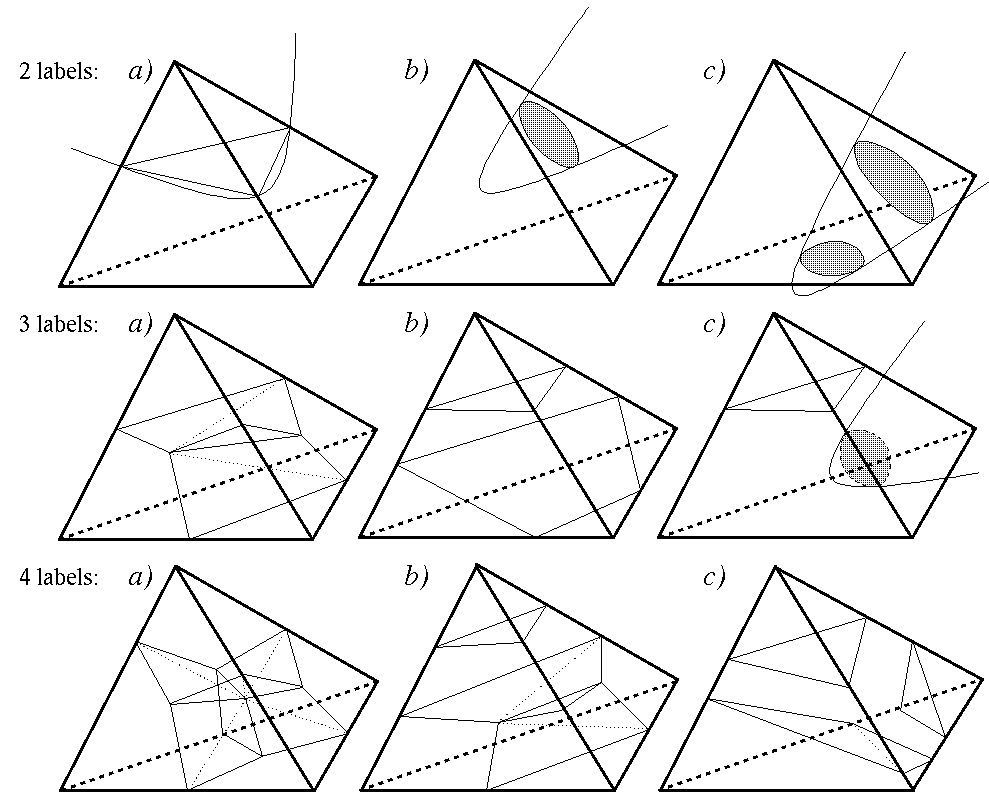Dealing with cell labels.

Determine which Voronoi regions intersect cell.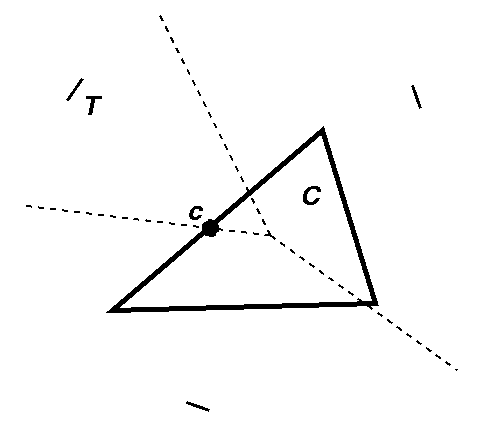Fact [Teichmann & Teller]: Voronoi region of t intersects cell C iff point closest to t on C is closer to t than any other site.

## Bounds

Worst-case complexity of the medial surface of a polyhedron?

Upper bound is O(n3+e).

Lower bound example with Omega(n2) faces: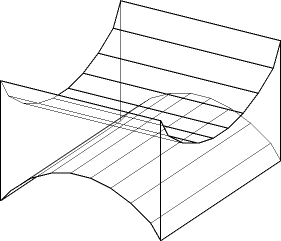Applications

## Motion Planning

Find path with large clearance to obstacles: [Overmars et al.]How to deal with non-point or non-circular objects?

## Collision Detection

Idea: For a pair of convex objects, keep track of pair of closest features. HOW?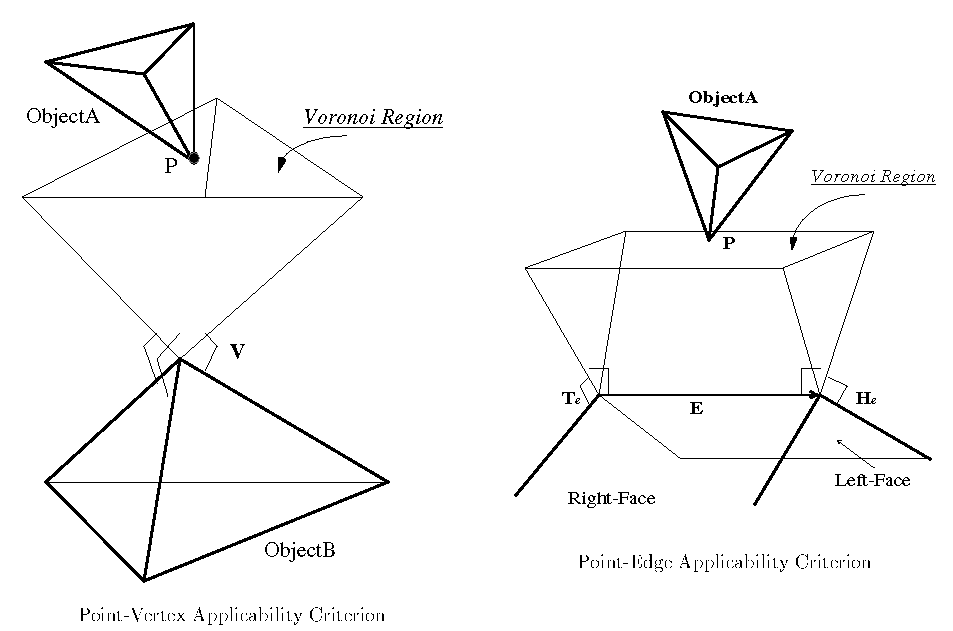Animation:Works only for convex objects. Generalization to non-convex objects.

## Nearest neighbor precomputation

Use with point location in Voronoi cells.

## Mesh generation.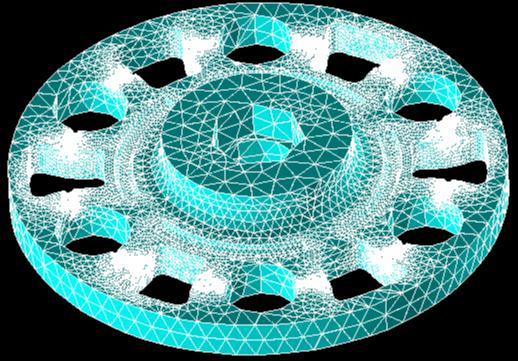## Skeletons for Animation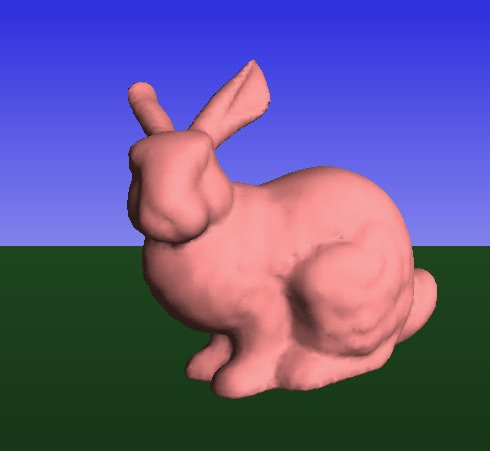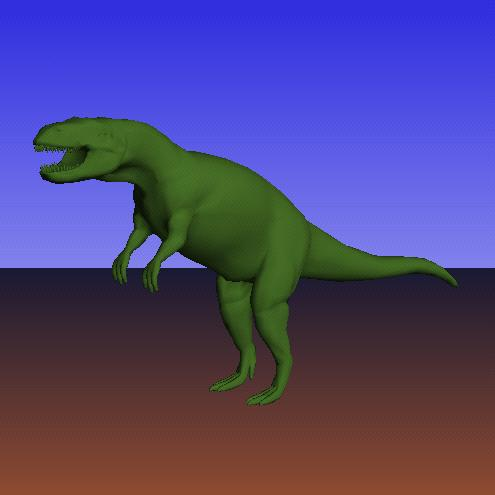<demo anim1, anim2>

The end.